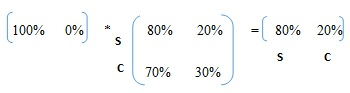# Markov chain and its use in solving real world problems

By Prateek Sharma & Priya Chetty on February 27, 2018

Markov chain is one of the most important tests in order to deal with independent trial processes. There are two major principal theorems for these processes. The first one is the ‘Law of Large Numbers’ and the second one is the ‘Central Limit Theorem’. When probability experiments create independent trial processes, the outcomes for all iterations are the same. Also, they increase or decrease with the same probability. Markov chain is the process where the outcome of a given experiment can affect the outcome of future experiments. It consists of a finite number of states and some known probabilities, where the probability of changing from state j to state i. For instance, there are two sectors; government and private. The probability here is the likelihood of moving from state j to state i in a given time for an individual.

Below are some examples of situations showing the application of the Markov chain.

## Markov chain application example 1

RA Howard explained Markov chain with the example of a frog in a pond jumping from lily pad to lily pad with the relative transition probabilities. Lily pads in the pond represent the finite states in the Markov chain and the probability is the odds of frog changing the lily pads.

## Markov chain application example 2

Manager in a company states their intention to get a promotion by the end of next month to her employee P. And P forwards the same message to Q, who further gives the news to R and so on. In such a scenario there is a probability x that a person will replace the answer from yes to no while conveying the message to the next person and a probability y that the person will change the answer from no to yes.

## Markov chain application example 3

Minister X has 55% votes and Minister Y has the rest 45% votes in one state. However, the probability of current followers voting Minister X staying with Minister X in the future is 70%, and switching to Minister Y is 30%. And the probability of the present followers voting Minister Y staying with Minister Y is 90% and switching to Minister X is 10%. Even though Minister X has more votes than Y in the current situation, but in the next election Minister Y will have more votes with 57% and X will be left with only 43% votes.

## The simple business case for the application of Markov chain

The case supposes that there are 2 types of weather in a particular area, ‘sunny’ and ‘cloudy’. A news channel wants to broadcast their prediction about the next week’s weather.

The news channel hires a weather forecast company to find out the next week’s weather and also for the following weeks. Currently, there is ‘sunny’ weather in that area.

• The probability of the weather staying ‘sunny’ the following week is 80%.
• Probability of the weather changing from ‘sunny’ to ‘cloudy’ over a week is 20%
• Probability of the weather staying ‘cloudy’ the following week is 70%
• Probability of the weather changing from ‘cloudy’ to ‘sunny’ over a week is 30%

Although the weather is predicted to be ‘sunny’ the whole week, one cannot be sure about the next week without making some transition calculations. The below matrix is to explain the transition:

### Transition diagram

The diagram simply shows the transitions and the weather conditions the same as the above matrix. The calculations can be done by the following matrix multiplication:

Current State * Transition Matrix = Final State

S=Sunny; C= CloudyIt can be seen that there is an 80% chance that the next whole week will also be ‘sunny’. But, there is a 20% chance that the weather might change to ‘cloudy’ next week. This calculation is called the Markov chain. If the transition matrix doesn’t change with time, one can also predict the weather for further weeks using the same equation. Calculations for the weather forecast in the two weeks’ time:Use 5E25A5EE63214 to save 5000 on 15001 - 20000 words standard order of literature survey.

Therefore, to predict the weather for further days/weeks/months in the long run, a steady-state vector helps in calculating the likelihood of the weather staying ‘sunny’ or changing to ‘cloudy’ without depending on the given initial condition of weather.

```Equation 1: 0.2x1 + 0.7x2 = 0 = 0.2x1 - 0.7x2
Equation 2: x1 + x2 = 1 (Probability vector)```

Therefore, from Equation 1 and 2, about 78% of the weeks in the future will be ‘sunny’, while the rest will be ‘cloudy’.

## Probable areas of application of Markov chain

Markov chain has many applications in the field of the real-world process are followings:-

• One of the most popular use of the Markov chain is in determining page rank by Google.
• Markov chain-based methods also used to efficiently compute integrals of high-dimensional functions.
• This method plays an important role to allow samples from any arbitrary probability distribution.
• It helps determine the probability of consumers switching from one brand to another.
• Markov chains are popular in finance and economics to model different phenomena, including market crashes and asset prices.

Software that can be used for Markov chain analysis, are Ram Commander, SoHaR Reliability and safety, Markov Analysis software, and MARCA (Markov Chain Analyzer).